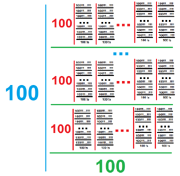11,053 Pages

The Pentfactor is equal to 100!5 using Extensible ! Notation. The term was coined by Yabuszko. This number is comparable to gigol and gorgegolVisual representation of pentfactor

## Etymology

The name of this number is based on Greek prefix "penta-" and the factor.

## Approximations

Notation Approximation
Arrow Notation $$82\uparrow\uparrow\uparrow\uparrow\uparrow101$$
Chained Arrow Notation $$82 \rightarrow 101 \rightarrow 5$$
BEAF $$\{82,101,5\}$$
Hyper-E notation

$$E160\#100\#\#4$$

Hyperfactorial Array Notation $$100!4$$
Strong Array Notation $$s(82,100,5)$$
Nested Factorial Notation $$100!$$
Fast-growing hierarchy $$f_6(100)$$
Hardy hierarchy $$H_{\omega^6}(100)$$
Slow-growing Hierarchy $$g_{\varphi(4,0)}(100)$$

## Sources

Community content is available under CC-BY-SA unless otherwise noted.